STAT 802

Assignment 1

1. Suppose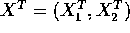has a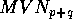distribution with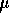partitioned as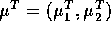and Variance covariance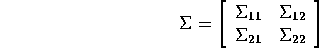1. Show that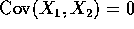if and only if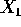and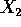are independent. Use my definition of MVN; you are not allowed to assume that X has a density. A mathematically careful argument my rely on the fact that if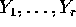are independent and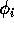are (measurable) functions then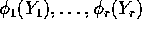are independent.
2. Show that whether or not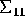is singular, each column of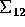is in the column space of.
3. Show that there is a matrix A which is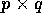such that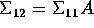.
4. Let h be a column ofand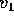and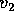be any vectors such that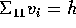for i=1,2. Show that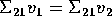.
5. Show that if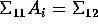for i=1,2 then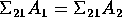.
6. Show that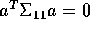implies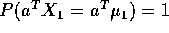for any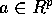.
7. Suppose x is such thatimplies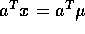. Show that the conditional distribution ofgiven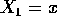is well-defined and is multivariate normal with mean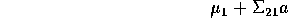and variance covariance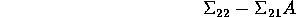where a is any solution of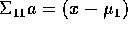and A is any solution of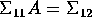.

NOTE: Most facts about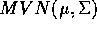variates X can be demonstrated by writing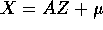for well chosen A.

2. Fix. Minimize and maximize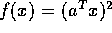subject to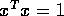.
3. Fix. Minimize and maximize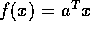subject to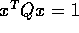for a given symmetric positive definite matrix Q.
4. Write out the spectral decomposition of the matrix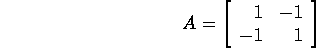and then find a symmetric square root.

5. Suppose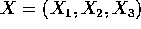has a MVN distribution with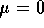andFind the conditional distribution ofgiven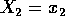and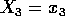. For which values of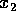and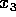does this make sense?

Richard Lockhart
Mon Jan 26 10:30:30 PST 1998